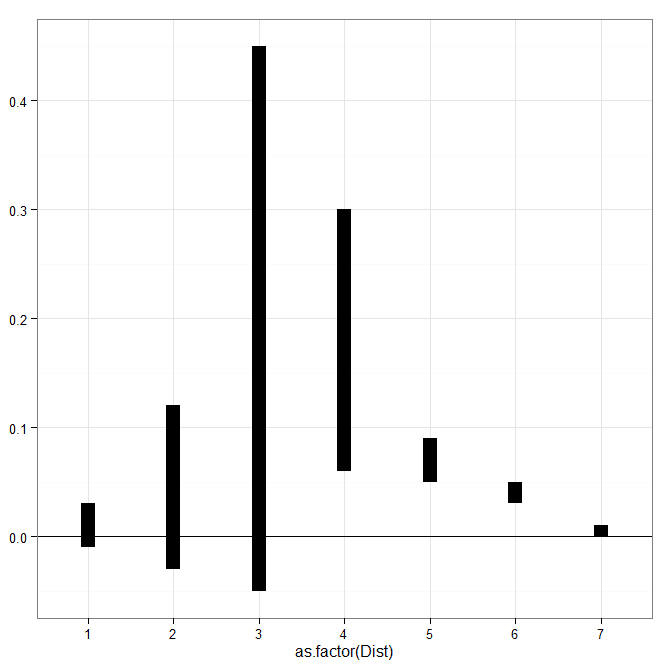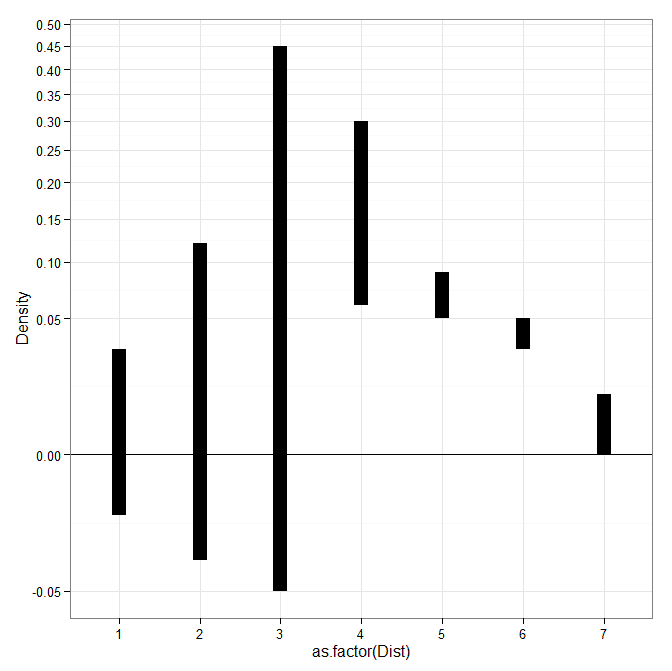Making a hexbin map in ggplot

In a recent working paper I made a hexbin map all in R. (Gio did most of the hard work of data munging and modeling though!) Figured I would detail the process here for some notes. Hexagon binning is purportedly better than regular squares (to avoid artifacts of runs in discretized data). But the reason I use them in this circumstance is mostly just an aesthetic preference.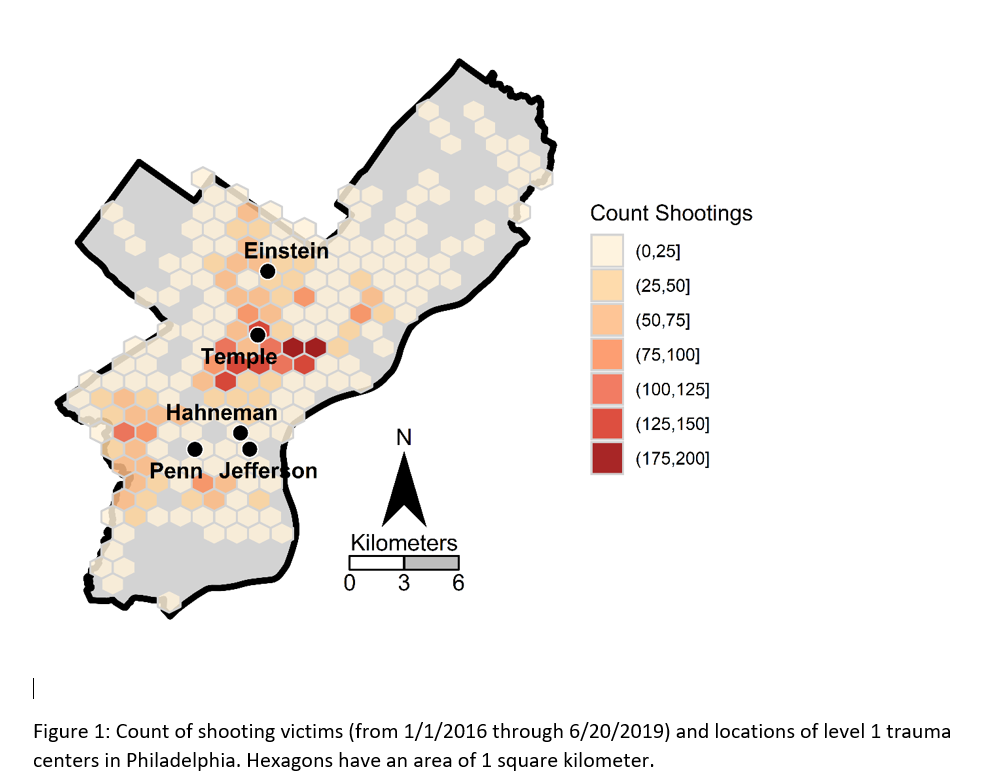Two tricky parts to this: 1) making the north arrow and scale bar, and 2) figuring out the dimensions to make regular hexagons. As an illustration I use the shooting victim data from Philly (see the working paper for all the details) full data and code to replicate here. I will walk through a bit of it though.

Data Prep

First to start out, I just use these three libraries, and set the working directory to where my data is.

library(ggplot2)
library(rgdal)
library(proj4)
setwd('C:\\Users\\axw161530\\Dropbox\\Documents\\BLOG\\HexagonMap_ggplot\\Analysis')

Now I read in the Philly shooting data, and then an outline of the city that is projected. Note I read in the shapefile data using rgdal, which imports the projection info. I need that to be able to convert the latitude/longitude spherical coordinates in the shooting data to a local projection. (Unless you are making a webmap, you pretty much always want to use some type of local projection, and not spherical coordinates.)

#Read in the shooting data
#Get rid of missing
shoot <- shoot[!is.na(shoot\$lng),c('lng','lat')]
#Read in the Philly outline
#Project the Shooting data
phill_pj <- proj4string(PhilBound)
XYMeters <- proj4::project(as.matrix(shoot[,c('lng','lat')]), proj=phill_pj)
shoot\$x <- XYMeters[,1]
shoot\$y <- XYMeters[,2]

Making a Basemap

It is a bit of work to make a nice basemap in R and ggplot, but once that upfront work is done then it is really easy to make more maps. To start, the GISTools package has a set of functions to get a north arrow and scale bar, but I have had trouble with them. The ggsn package imports the north arrow as a bitmap instead of vector, and I also had a difficult time with its scale bar function. (I have not figured out the cartography package either, I can’t keep up with all the mapping stuff in R!) So long story short, this is my solution to adding a north arrow and scale bar, but I admit better solutions probably exist.

So basically I just build my own polygons and labels to add into the map where I want. Code is motivated based on the functions in GISTools.

#creating north arrow and scale bar, motivation from GISTools package
arrow_data <- function(xb, yb, len) {
s <- len
arrow.x = c(0,0.5,1,0.5,0) - 0.5
arrow.y = c(0,1.7  ,0,0.5,0)
adata <- data.frame(aX = xb + arrow.x * s, aY = yb + arrow.y * s)
}

scale_data <- function(llx,lly,len,height){
box1 <- data.frame(x = c(llx,llx+len,llx+len,llx,llx),
y = c(lly,lly,lly+height,lly+height,lly))
box2 <- data.frame(x = c(llx-len,llx,llx,llx-len,llx-len),
y = c(lly,lly,lly+height,lly+height,lly))
return(list(box1,box2))
}

x_cent <- 830000
len_bar <- 3000
offset_scaleNum <- 64300
arrow <- arrow_data(xb=x_cent,yb=67300,len=2500)
scale_bxs <- scale_data(llx=x_cent,lly=65000,len=len_bar,height=750)

lab_data <- data.frame(x=c(x_cent, x_cent-len_bar, x_cent, x_cent+len_bar, x_cent),
y=c( 72300, offset_scaleNum, offset_scaleNum, offset_scaleNum, 66500),
lab=c("N","0","3","6","Kilometers"))

This is about the best I have been able to automate the creation of the north arrow and scale bar polygons, while still having flexibility where to place the labels. But now we have all of the ingredients necessary to make our basemap. Make sure to use coord_fixed() for maps! Also for background maps I typically like making the outline thicker, and then have borders for smaller polygons lighter and thinner to create a hierarchy. (If you don’t want the background map to have any color, use fill=NA.)

base_map <- ggplot() +
geom_polygon(data=PhilBound,size=1.5,color='black', fill='darkgrey', aes(x=long,y=lat)) +
geom_polygon(data=arrow, fill='black', aes(x=aX, y=aY)) +
geom_polygon(data=scale_bxs[], fill='grey', color='black', aes(x=x, y = y)) +
geom_polygon(data=scale_bxs[], fill='white', color='black', aes(x=x, y = y)) +
geom_text(data=lab_data, size=4, aes(x=x,y=y,label=lab)) +
coord_fixed() + theme_void()

#Check it out
base_map

This is what it looks like on my windows machine in RStudio — it ends up looking alittle different when I export the figure straight to PNG though. Will get to that in a minute.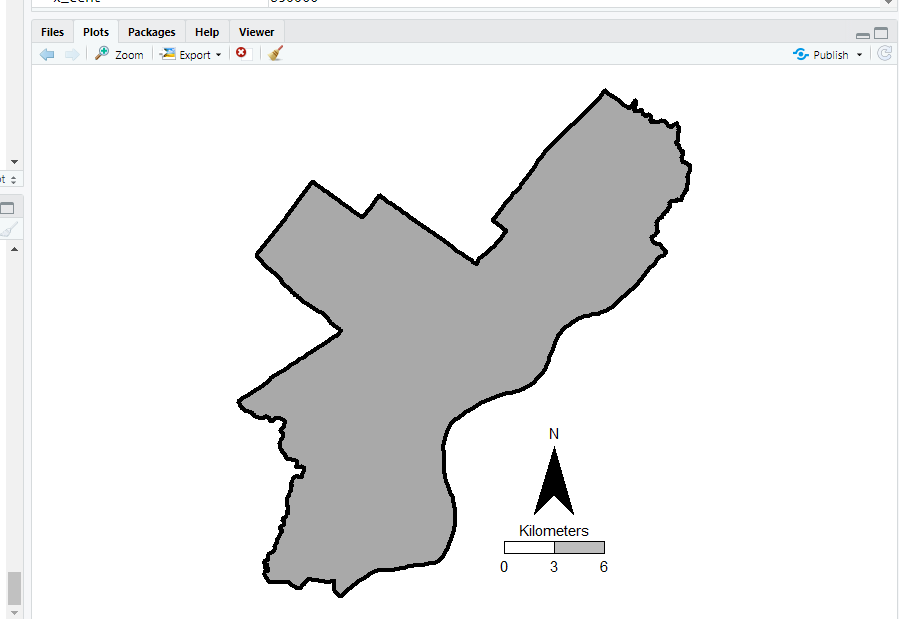Making a hexagon map

Now you have your basemap you can superimpose whatever other data you want. Here I wanted to visualize the spatial distribution of shootings in Philly. One option is a kernel density map. I tend to like aggregated count maps though better for an overview, since I don’t care so much for drilling down and identifying very specific hot spots. And the counts are easier to understand than densities.

In geom_hex you can supply a vertical and horizontal parameter to control the size of the hexagon — supplying the same for each does not create a regular hexagon though. The way the hexagon is oriented in geom_hex the vertical parameter is vertex to vertex, whereas the horizontal parameter is side to side.

Here are three helper functions. First, wd_hex gives you a horizontal width length given the vertical parameter. So if you wanted your hexagon to be vertex to vertex to be 1000 meters (so a side is 500 meters), wd_hex(1000) returns just over 866. Second, if for your map you wanted to convert the numbers to densities per unit area, you can use hex_area to figure out the size of your hexagon. Going again with our 1000 meters vertex to vertex hexagon, we have a total of hex_area(1000/2) is just under 650,000 square meters (or about 0.65 square kilometers).

For maps though, I think it makes the most sense to set the hexagon to a particular area. So hex_dim does that. If you want to set your hexagons to a square kilometer, given our projected data is in meters, we would then just do hex_dim(1000^2), which with rounding gives us vert/horz measures of about (1241,1075) to supply to geom_hex.

#ggplot geom_hex you need to supply height and width
#if you want a regular hexagon though, these
#are not equal given the default way geom_hex draws them
#https://www.varsitytutors.com/high_school_math-help/how-to-find-the-area-of-a-hexagon

#get width given height
wd_hex <- function(height){
tri_side <- height/2
sma_side <- height/4
width <- 2*sqrt(tri_side^2 - sma_side^2)
return(width)
}

#now to figure out the area if you want
#side is simply height/2 in geom_hex
hex_area <- function(side){
area <- 6 * (  (sqrt(3)*side^2)/4 )
return(area)
}

#So if you want your hexagon to have a regular area need the inverse function
#Gives height and width if you want a specific area
hex_dim <- function(area){
num <- 4*area
den <- 6*sqrt(3)
vert <- 2*sqrt(num/den)
horz <- wd_hex(height)
return(c(vert,horz))
}

my_dims <- hex_dim(1000^2)   #making it a square kilometer
sqrt(hex_area(my_dims/2)) #check to make sure it is square km
#my_dims also checks out with https://hexagoncalculator.apphb.com/

Now onto the good stuff. I tend to think discrete bins make nicer looking maps than continuous fills. So through some trial/error you can figure out the best way to make those via cut. Also I make the outlines for the hexagons thin and white, and make the hexagons semi-transparent. So you can see the outline for the city. I like how by default areas with no shootings are not given any hexagon.

lev_cnt <- seq(0,225,25)
shoot_count <- base_map +
geom_hex(data=shoot, color='white', alpha=0.85, size=0.1, binwidth=my_dims,
aes(x=x,y=y,fill=cut(..count..,lev_cnt))) +
scale_fill_brewer(name="Count Shootings", palette="OrRd")

We have come so far, now to automate exporting the figure to a PNG file. I’ve had trouble getting journals recently to not bungle vector figures that I forward them, so I am just like going with high res PNG to avoid that hassle. If you render the figure and use the GUI to export to PNG, it won’t be as high resolution, so you can often easily see aliasing pixels (e.g. the pixels in the North Arrow for the earlier base map image).

png('Philly_ShootCount.png', height=5, width=5, units="in", res=1000, type="cairo")
shoot_count
dev.off()

Note the font size/location in the exported PNG are often not quite exactly as they are when rendered in the RGUI window or RStudio on my windows machine. So make sure to check the PNG file.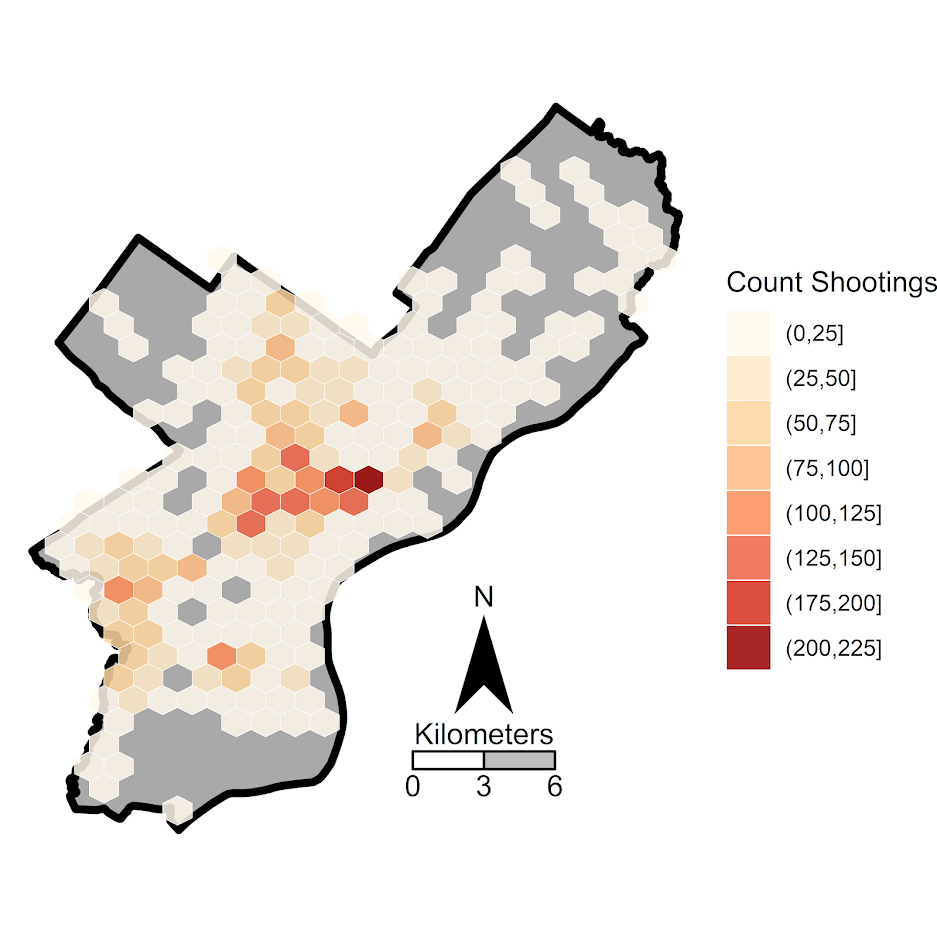Making interactive plots with R and Plotly

I wrote a small op-ed based on the homicide studies work I recently published about interpreting crime trends. Unfortunately that op-ed was not picked up by anyone (I missed the timing abit, maybe next year when the UCR stats come out I can just update the numbers and make the same point). I’ve posted that op-ed here, and I wanted to make a quick blog post detailing how I made the interactive graphs in that post using R and the Plotly library. All the data and code to replicate this can be downloaded from here.

Unfortunately with my free wordpress blog I cannot embed the actual interactive graphics, but I will provide links to online versions at my UT Dallas page that work and show a screenshot of each. So first, lets load all of the libraries that you will need, as well as set the working directory. (Of course change it to where you have your data saved on your local machine.)

#########################################################
#Making a shiny app for homicide rate chart
library(shiny)
library(ggplot2)
library(plotly)
library(htmlwidgets)
library(scales)

mydir <- "C:\\Users\\axw161530\\Box Sync\\Projects\\HomicideGraphs\\Analysis\\Analysis"
setwd(mydir)
#########################################################

Now I just read in the data. I have two datasets, the funnel rates just has additional columns to draw the funnel graphs already created. (See here or here, or the data in the original Homicide Studies paper linked at the top, on how to construct these.)

############################################################
#Get the data

summary(FunnRates)
FunnRates\$Population <- FunnRates\$Pop1 #These are just to make nicer labels
FunnRates\$HomicideRate <- FunnRates\$HomRate

summary(IntRates)
############################################################

Funnel Chart for One Year

First, plotly makes it dead easy to take graphs you created via ggplot and turn them into an interactive graph. So here is a link to the interactive chart, and below is a screenshot.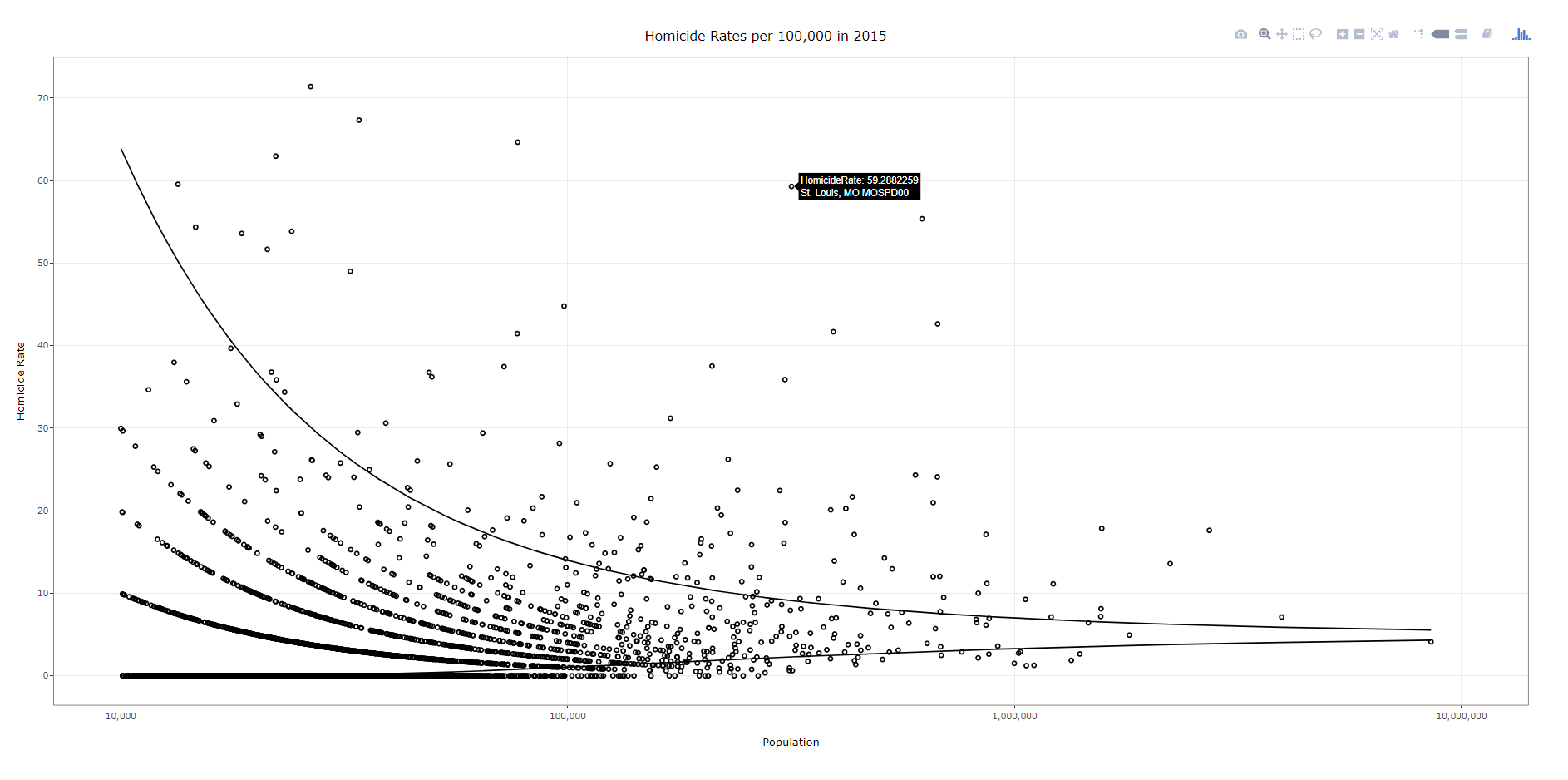To walk through the code, first you make your (almost) plane Jane ggplot object. Here I name it p. You will get an error for an “unknown aesthetics: text”, but this will be used by plotly to create tooltips. Then you use the ggplotly function to turn the original ggplot graph p into an interactive graph. By default the plotly object has more stuff in the tooltip than I want, which you can basically just go into the innards of the plotly object and strip out. Then the final part is just setting the margins to be alittle larger than default, as the axis labels were otherwise slightly cut-off.

############################################################
#Make the funnel chart
year_sel <- 2015
p <- ggplot(data = FunnRates[FunnRates\$Year == year_sel,]) + geom_point(aes(x=Population, y=HomicideRate, text=NiceLab), pch=21) +
geom_line(aes(x=Population,y=LowLoc99)) + geom_line(aes(x=Population,y=HighLoc99)) +
labs(title = paste0("Homicide Rates per 100,000 in ",year_sel)) +
scale_x_log10("Population", limits=c(10000,10000000), breaks=c(10^4,10^5,10^6,10^7), labels=comma) +
scale_y_continuous("Homicide Rate", breaks=seq(0,110,10)) +
theme_bw() #+ theme(text = element_text(size=20), axis.title.y=element_text(margin=margin(0,10,0,0)))

pl <- ggplotly(p, tooltip = c("HomicideRate","text"))
#pl <- plotly_build(p, width=1000, height=900)
#See https://stackoverflow.com/questions/45801389/disable-hover-information-for-a-specific-layer-geom-of-plotly
pl\$x\$data[]\$hoverinfo <- "none"
pl\$x\$data[]\$hoverinfo <- "none"
pl <- pl %>% layout(margin = list(l = 75, b = 65))
############################################################

After this point you can just type pl into the console and it will open up an interactive window. Or you can use the saveWidget function from the htmlwidgets package, something like saveWidget(as_widget(pl), "FunnelChart_2015.html", selfcontained=TRUE) to save the graph to an html file.

Now there are a couple of things. You can edit various parts of the graph, such as its size and label text size, but depending on your application these might not be a good idea. If you need to take into account smaller screens, I think it is best to use some of the defaults, as they adjust per the screen that is in use. For the size of the graph if you are embedding it in a webpage using iframe’s you can set the size at that point. If you look at my linked op-ed you can see I make the funnel chart taller than wider — that is through the iframe specs.

Funnel Chart over Time

Ok, now onto the fun stuff. So we have a funnel chart for one year, but I have homicide years from 1965 through 2015. Can we examine those over time. Plotly has an easy to use additional argument to ggplot graphs, named Frame, that allows you to add a slider to the interactive chart for animation. The additional argument ids links one object over time, ala Hans Rosling bubble chart over time. Here is a link to the interactive version, and below is a screen shot: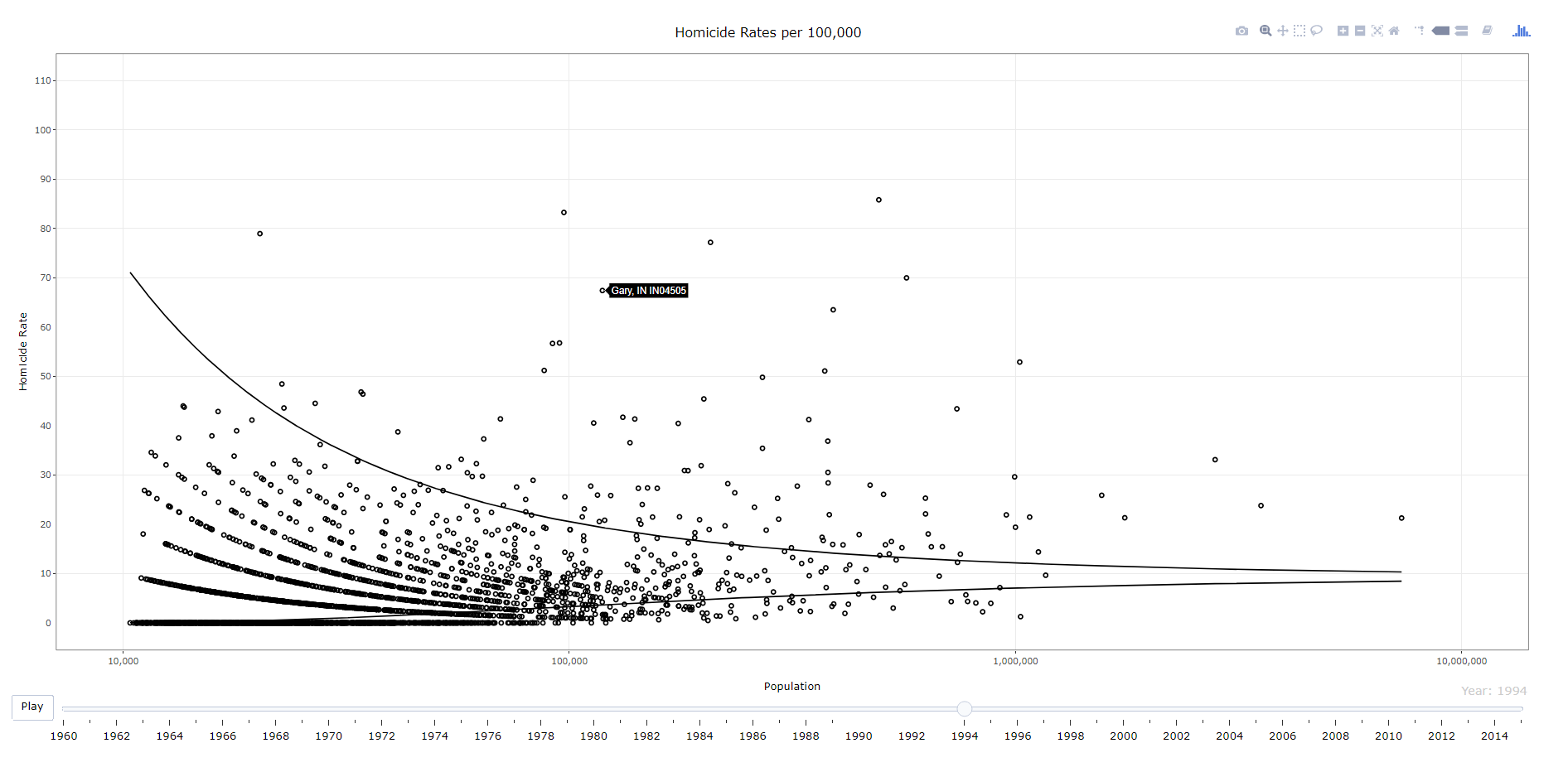############################################################
#Making the funnel chart where you can select the year
py <- ggplot(data = FunnRates) + geom_point(aes(x=Population, y=HomicideRate, text=NiceLab, frame=Year,ids=ORI), pch=21) +
geom_line(aes(x=Population,y=LowLoc99,frame=Year)) + geom_line(aes(x=Population,y=HighLoc99,frame=Year)) +
labs(title = paste0("Homicide Rates per 100,000")) +
scale_x_log10("Population", limits=c(10000,10000000), breaks=c(10^4,10^5,10^6,10^7), labels=comma) +
scale_y_continuous("Homicide Rate", breaks=seq(0,110,10), limits=c(0,110)) +
theme_bw() #+ theme(text = element_text(size=20), axis.title.y=element_text(margin=margin(0,10,0,0)))

ply <- ggplotly(py, tooltip = c("text")) %>% animation_opts(0, redraw=FALSE)
ply\$x\$data[]\$hoverinfo <- "none"
ply\$x\$data[]\$hoverinfo <- "none"
saveWidget(as_widget(ply), "FunnelChart_YearSelection.html", selfcontained=FALSE)
############################################################

The way I created the data it does not make sense to do a smooth animation for the funnel line, so this just flashes to each new year (via the animation_opts spec). (I could make the data so it would look nicer in an animation, but will wait for someone to pick up the op-ed before I bother too much more with this.) But it accomplishes via the slider the ability for you to pick which year you want.

Fan Chart Just One City

Next we are onto the fan charts for each individual city with the prediction intervals. Again you can just create this simple chart in ggplot, and then use plotly to make a version with tooltips. Here is a link to an interactive version, and below is a screenshot.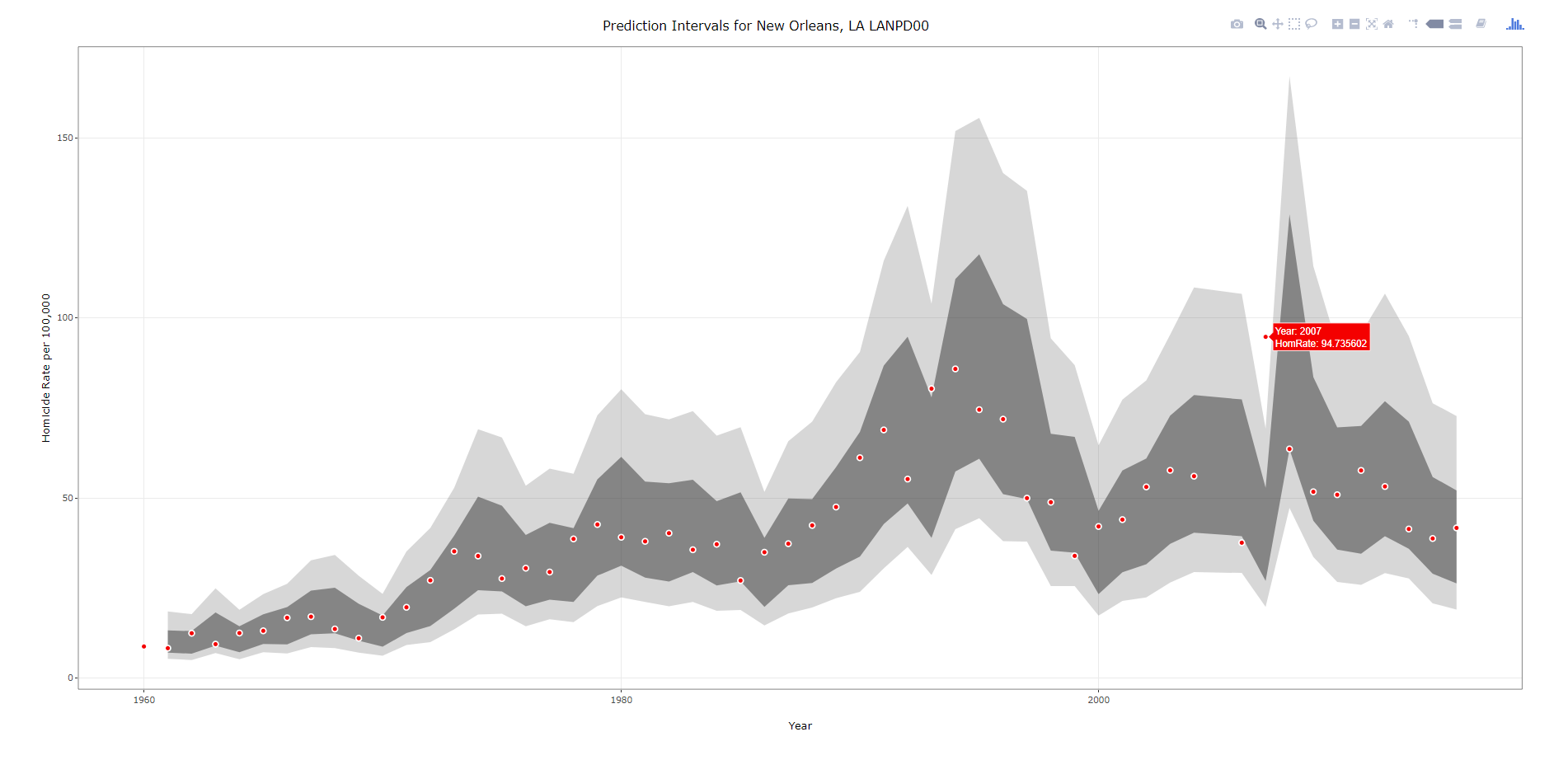###################################################
#Making the fan graph for New Orleans
titleLab <- unique(IntRates[,c("ORI","NiceLab","AgencyName","State")])
p2 <- ggplot(data=IntRates[IntRates\$ORI == "LANPD00",], aes(x=Year, y=HomRate)) +
geom_ribbon(aes(ymin=LowB, ymax=HighB), alpha=0.2) +
geom_ribbon(aes(ymin=LagLow25, ymax=LagHigh25), alpha=0.5) +
geom_point(shape=21, color="white", fill="red", size=2) +
labs(x = "Year", y="Homicide Rate per 100,000") +
#scale_x_continuous(breaks=seq(1960,2015,by=5)) +
ggtitle(paste0("Prediction Intervals for ",titleLab[titleLab\$ORI == "LANPD00",c("NiceLab")])) +
theme_bw() #+ theme(text = element_text(size=20), axis.title.y=element_text(margin=margin(0,10,0,0)))
#p2
pl2 <- ggplotly(p2, tooltip = c("Year","HomRate"), dynamicTicks=TRUE)
pl2\$x\$data[]\$hoverinfo <- "none"
pl2\$x\$data[]\$hoverinfo <- "none"
pl2 <- pl2 %>% layout(margin = list(l = 100, b = 65))
#pl2
saveWidget(as_widget(pl2), "FanChart_NewOrleans.html", selfcontained=FALSE)
###################################################

Note when you save the widget to selfcontained=FALSE, it hosts several parts of the data into separate folders. I always presumed this was more efficient than making one huge html file, but I don’t know for sure.

Fan Chart with Dropdown Selection

Unfortunately the frame type animation does not make as much sense here. It would be hard for someone to find a particular city of interest in that slider (as a note though the slider can have nominal data, if I only had a few cities it would work out ok, with a few hundred it will not though). So feature request if anyone from plotly is listening — please have a dropdown type option for ggplot graphs! In the meantime though there is an alternative using a tradition plot_ly type chart. Here is that interactive fan chart with a police agency dropdown, and below is a screenshot.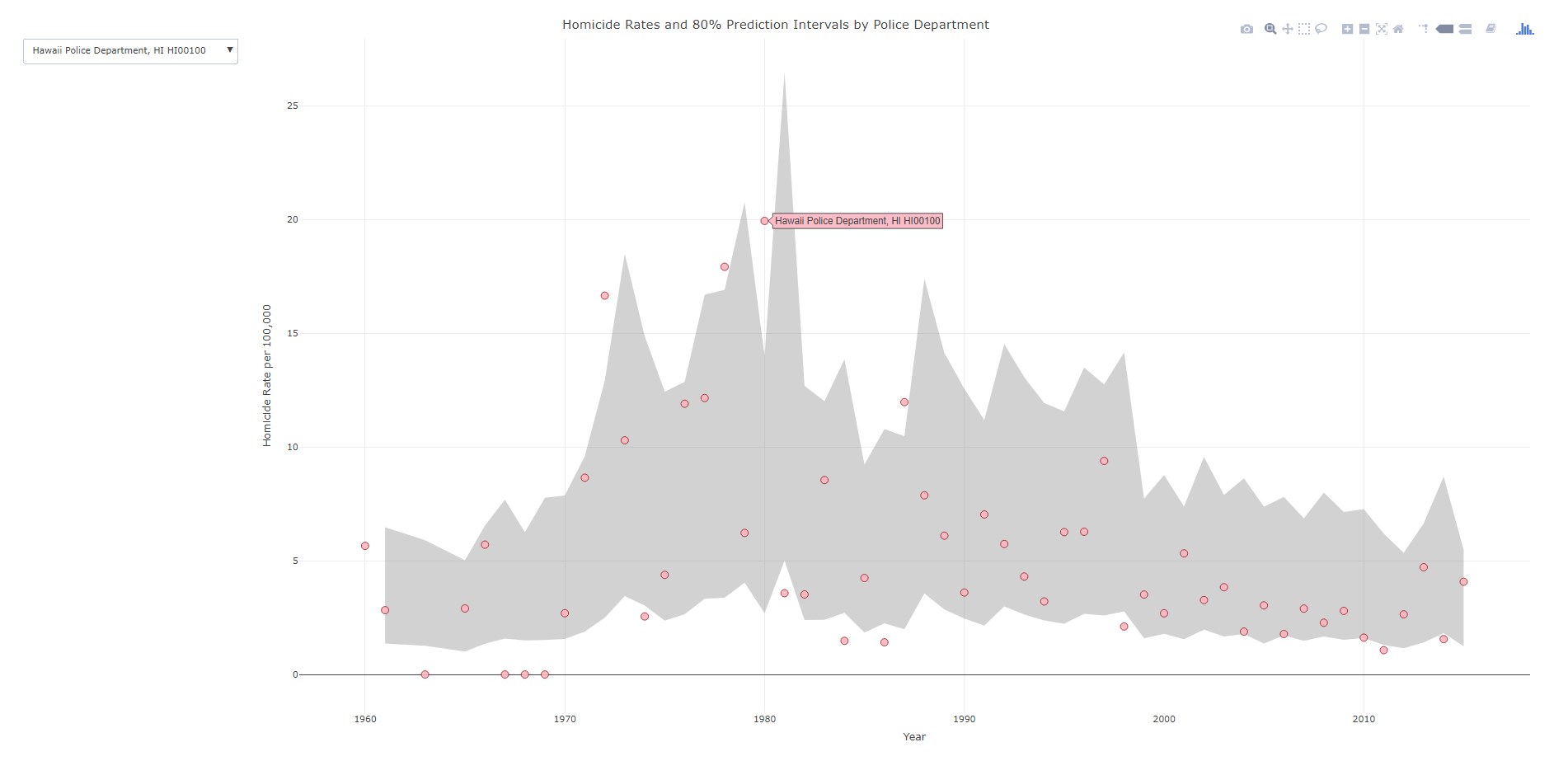###################################################
#Making the fan graph where you can select the city of interest
#Need to have a dropdown for the city

titleLab <- unique(IntRates[,c("ORI","NiceLab","State")])
nORI <- length(titleLab[,1])
choiceP <- vector("list",nORI)
for (i in 1:nORI){
choiceP[[i]] <- list(method="restyle", args=list("transforms.value", unique(IntRates\$NiceLab)[i]), label=titleLab[i,c("NiceLab")])
}

trans <- list(list(type='filter',target=~NiceLab, operation="=", value=unique(IntRates\$NiceLab)))
textLab <- ~paste("Homicide Rate:",HomRate,'\$
Year:',Year,'\$
Homicides:',Homicide,'\$
Population:',Pop1,'\$
Agency Name:',NiceLab)

#Lets try with the default plotly
#See https://community.plot.ly/t/need-help-on-using-dropdown-to-filter/6596
ply4 <- IntRates %>%
plot_ly(x= ~Year,y= ~HighB, type='scatter', mode='lines', line=list(color='transparent'), showlegend=FALSE, name="90%", hoverinfo="none", transforms=trans) %>%
add_trace(y=~LowB,  type='scatter', mode='lines', line=list(color='transparent'), showlegend=FALSE, name='10%', hoverinfo="none", transforms=trans,
fill = 'tonexty', fillcolor='rgba(105,105,105,0.3)') %>%
add_trace(x=~Year,y=~HomRate, text=~NiceLab, type='scatter', mode='markers', marker = list(size=10, color = 'rgba(255, 182, 193, .9)', line = list(color = 'rgba(152, 0, 0, .8)', width = 1)),
hoverinfo='text', text=textLab, transforms=trans) %>%
layout(title = "Homicide Rates and 80% Prediction Intervals by Police Department",
xaxis = list(title="Year"),
yaxis = list(title="Homicide Rate per 100,000"),

saveWidget(as_widget(ply4), "FanChart_Dropdown.html", selfcontained=FALSE)
###################################################

So in short plotly makes it super-easy to make interactive graphs with tooltips. Long term goal I would like to make a visual supplement to the traditional UCR report (I find the complaint of what tables to include to miss the point — there are much better ways to show the information that worrying about the specific tables). So if you would like to work on that with me always feel free to get in touch!

Some plots to go with group based trajectory models in R

On my prior post on estimating group based trajectory models in R using the crimCV package I received a comment asking about how to plot the trajectories. The crimCV model object has a base plot object, but here I show how to extract those model predictions as well as some other functions. Many of these plots are illustrated in my paper for crime trajectories at micro places in Albany (forthcoming in the Journal of Quantitative Criminology). First we are going to load the crimCV and the ggplot2 package, and then I have a set of four helper functions which I will describe in more detail in a minute. So run this R code first.

library(crimCV)
library(ggplot2)

long_traj <- function(model,data){
df <- data.frame(data)
vars <- names(df)
prob <- model['gwt'] #posterior probabilities
df\$GMax <- apply(prob\$gwt,1,which.max) #which group # is the max
df\$PMax <- apply(prob\$gwt,1,max)       #probability in max group
df\$Ord <- 1:dim(df)                 #Order of the original data
prob <- data.frame(prob\$gwt)
names(prob) <- paste0("G",1:dim(prob)) #Group probabilities are G1, G2, etc.
longD <- reshape(data.frame(df,prob), varying = vars, v.names = "y",
timevar = "x", times = 1:length(vars),
direction = "long") #Reshape to long format, time is x, y is original count data
return(longD)                        #GMax is the classified group, PMax is the probability in that group
}

weighted_means <- function(model,long_data){
G_names <- paste0("G",1:model\$ng)
G <- long_data[,G_names]
W <- G*long_data\$y                                    #Multiple weights by original count var
Agg <- aggregate(W,by=list(x=long_data\$x),FUN="sum")  #then sum those products
mass <- colSums(model\$gwt)                            #to get average divide by total mass of the weight
for (i in 1:model\$ng){
Agg[,i+1] <- Agg[,i+1]/mass[i]
}
long_weight <- reshape(Agg, varying=G_names, v.names="w_mean",
timevar = "Group", times = 1:model\$ng,
direction = "long")           #reshape to long
return(long_weight)
}

pred_means <- function(model){
prob <- model\$prob               #these are the model predicted means
Xb <- model\$X %*% model\$beta     #see getAnywhere(plot.dmZIPt), near copy
lambda <- exp(Xb)                #just returns data frame in long format
p <- exp(-model\$tau * t(Xb))
p <- t(p)
p <- p/(1 + p)
mu <- (1 - p) * lambda
t <- 1:nrow(mu)
myDF <- data.frame(x=t,mu)
long_pred <- reshape(myDF, varying=paste0("X",1:model\$ng), v.names="pred_mean",
timevar = "Group", times = 1:model\$ng, direction = "long")
return(long_pred)
}

#Note, if you estimate a ZIP model instead of the ZIP-tau model
#use this function instead of pred_means
pred_means_Nt <- function(model){
prob <- model\$prob               #these are the model predicted means
Xb <- model\$X %*% model\$beta     #see getAnywhere(plot.dmZIP), near copy
lambda <- exp(Xb)                #just returns data frame in long format
Zg <- model\$Z %*% model\$gamma
p <- exp(Zg)
p <- p/(1 + p)
mu <- (1 - p) * lambda
t <- 1:nrow(mu)
myDF <- data.frame(x=t,mu)
long_pred <- reshape(myDF, varying=paste0("X",1:model\$ng), v.names="pred_mean",
timevar = "Group", times = 1:model\$ng, direction = "long")
return(long_pred)
}

occ <- function(long_data){
subdata <- subset(long_data,x==1)
agg <- aggregate(subdata\$PMax,by=list(group=subdata\$GMax),FUN="mean")
names(agg) <- "AvePP" #average posterior probabilites
agg\$Freq <- as.data.frame(table(subdata\$GMax))[,2]
n <- agg\$AvePP/(1 - agg\$AvePP)
p <- agg\$Freq/sum(agg\$Freq)
d <- p/(1-p)
agg\$OCC <- n/d #odds of correct classification
agg\$ClassProp <- p #observed classification proportion
#predicted classification proportion
agg\$PredProp <- colSums(as.matrix(subdata[,grep("^[G][0-9]", names(subdata), value=TRUE)]))/sum(agg\$Freq)
#Jeff Ward said I should be using PredProb instead of Class prop for OCC
agg\$occ_pp <- n/ (agg\$PredProp/(1-agg\$PredProp))
return(agg)
}

Now we can just use the data in the crimCV package to run through an example of a few different types of plots. First lets load in the TO1adj data, estimate the group based model, and make our base plot.

plot(out1)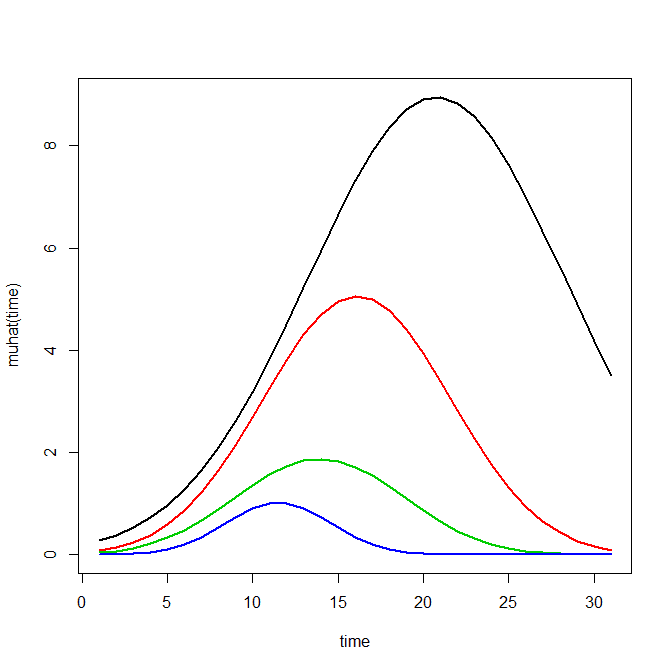Now most effort seems to be spent on using model selection criteria to pick the number of groups, what may be called relative model comparisons. Once you pick the number of groups though, you should still be concerned with how well the model replicates the data at hand, e.g. absolute model comparisons. The graphs that follow help assess this. First we will use our helper functions to make three new objects. The first function, long_traj, takes the original model object, out1, as well as the original matrix data used to estimate the model, TO1adj. The second function, weighted_means, takes the original model object and then the newly created long_data longD. The third function, pred_means, just takes the model output and generates a data frame in wide format for plotting (it is the same underlying code for plotting the model).

x <- weighted_means(model=out1,long_data=longD)
pred <- pred_means(model=out1)

We can subsequently use the long data longD to plot the individual trajectories faceted by their assigned groups. I have an answer on cross validated that shows how effective this small multiple design idea can be to help disentangle complicated plots.

#plot of individual trajectories in small multiples by group
p <- ggplot(data=longD, aes(x=x,y=y,group=Ord)) + geom_line(alpha = 0.1) + facet_wrap(~GMax)
p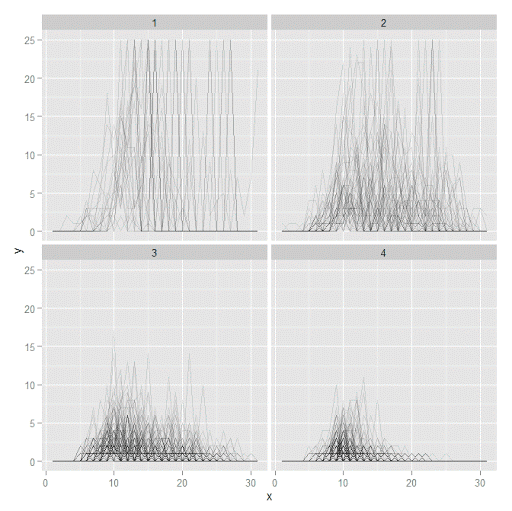Plotting the individual trajectories can show how well they fit the predicted model, as well as if there are any outliers. You could get more fancy with jittering (helpful since there is so much overlap in the low counts) but just plotting with a high transparency helps quite abit. This second graph plots the predicted means along with the weighted means. What the weighted_means function does is use the posterior probabilities of groups, and then calculates the observed group averages per time point using the posterior probabilities as the weights.

#plot of predicted values + weighted means
p2 <- ggplot() + geom_line(data=pred, aes(x=x,y=pred_mean,col=as.factor(Group))) +
geom_line(data=x, aes(x=x,y=w_mean,col=as.factor(Group))) +
geom_point(data=x, aes(x=x,y=w_mean,col=as.factor(Group)))
p2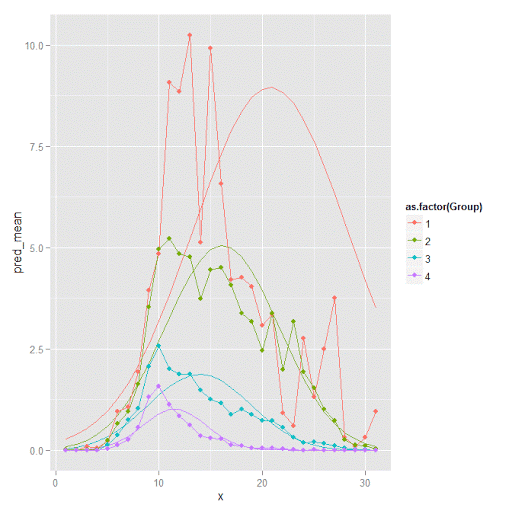Here you can see that the estimated trajectories are not a very good fit to the data. Pretty much eash series has a peak before the predicted curve, and all of the series except for 2 don’t look like very good candidates for polynomial curves.

It ends up that often the weighted means are very nearly equivalent to the unweighted means (just aggregating means based on the classified group). In this example the predicted values are a colored line, the weighted means are a colored line with superimposed points, and the non-weighted means are just a black line.

#predictions, weighted means, and non-weighted means
nonw_means <- aggregate(longD\$y,by=list(Group=longD\$GMax,x=longD\$x),FUN="mean")
names(nonw_means) <- "y"

p3 <- p2 + geom_line(data=nonw_means, aes(x=x,y=y), col='black') + facet_wrap(~Group)
p3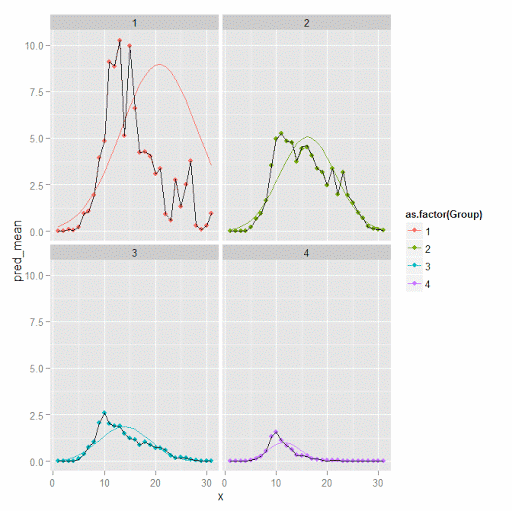You can see the non-weighted means are almost exactly the same as the weighted ones. For group 3 you typically need to go to the hundredths to see a difference.

#check out how close
nonw_means[nonw_means\$Group==3,'y'] -  x[x\$Group==3,'w_mean']

You can subsequently superimpose the predicted group means over the individual trajectories as well.

#superimpose predicted over ind trajectories
pred\$GMax <- pred\$Group
p4 <- ggplot() + geom_line(data=pred, aes(x=x,y=pred_mean), col='red') +
geom_line(data=longD, aes(x=x,y=y,group=Ord), alpha = 0.1) + facet_wrap(~GMax)
p4Two types of absolute fit measures I’ve seen advocated in the past are the average maximum posterior probability per group and the odds of correct classification. The occ function calculates these numbers given two vectors (one of the max probabilities and the other of the group classifications). We can get this info from our long data by just selecting a subset from one time period. Here the output at the console shows that we have quite large average posterior probabilities as well as high odds of correct classification. (Also updated to included the observed classified proportions and the predicted proportions based on the posterior probabilities. Again, these all show very good model fit.) Update: Jeff Ward sent me a note saying I should be using the predicted proportion in each group for the occ calculation, not the assigned proportion based on the max. post. prob. So I have updated to include the occ_pp column for this, but left the old occ column in as a paper trail of my mistake.

occ(longD)
#  group     AvePP Freq        OCC  ClassProp   PredProp     occ_pp
#1     1 0.9880945   23 1281.00444 0.06084656 0.06298397 1234.71607
#2     2 0.9522450   35  195.41430 0.09259259 0.09005342  201.48650
#3     3 0.9567524   94   66.83877 0.24867725 0.24936266   66.59424
#4     4 0.9844708  226   42.63727 0.59788360 0.59759995   42.68760

A plot to accompany this though is a jittered dot plot showing the maximum posterior probability per group. You can here that groups 3 and 4 are more fuzzy, whereas 1 and 2 mostly have very high probabilities of group assignment.

#plot of maximum posterior probabilities
subD <- longD[x==1,]
p5 <- ggplot(data=subD, aes(x=as.factor(GMax),y=PMax)) + geom_point(position = "jitter", alpha = 0.2)
p5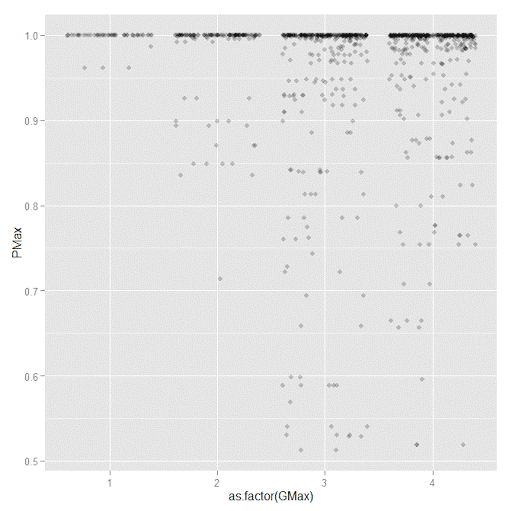Remember that these latent class models are fuzzy classifiers. That is each point has a probability of belonging to each group. A scatterplot matrix of the individual probabilities will show how well the groups are separated. Perfect separation into groups will result in points hugging along the border of the graph, and points in the middle suggest ambiguity in the class assignment. You can see here that each group closer in number has more probability swapping between them.

#scatterplot matrix
library(GGally)
sm <- ggpairs(data=subD, columns=4:7)
sm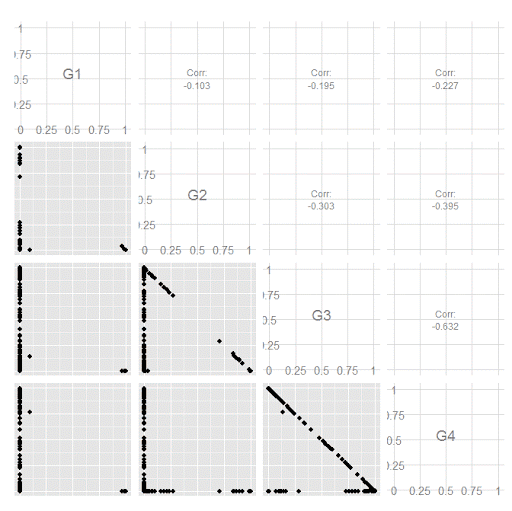And the last time series plot I have used previously is a stacked area chart.

#stacked area chart
nonw_sum <- aggregate(longD\$y,by=list(Group=longD\$GMax,x=longD\$x),FUN="sum")
names(nonw_sum) <- "y"
p6 <- ggplot(data=nonw_sum, aes(x=x,y=y,fill=as.factor(Group))) + geom_area(position='stack')
p6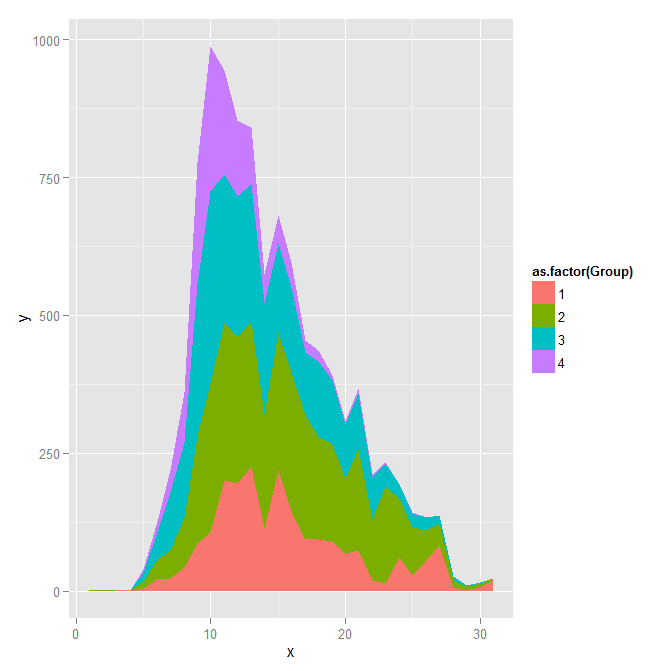I will have to put another post in the queue to talk about the spatial point pattern tests I used in that trajectory paper for the future as well.

Custom square root scale (with negative values) in ggplot2 (R)

My prior rootogram post Jon Peck made the astute comment that rootograms typically are plotted on a square root scale. (Which should of been obvious to me given the name!) The reason for a square root scale for rootograms is visualization purposes, the square root scale gives more weight to values nearby 0 and shrinks values farther away from 0.

SPSS can not have negative values on a square root scale, but you can make a custom scale using ggplot2 and the scales package in R for this purpose. Here I just mainly replicated this short post by Paul Hiemstra.

So in R, first we load the scales and the ggplot2 package, and then create our custom scale function. Obviously the square root of a negative value is not defined for real numbers, so what we do is make a custom square root function. The function simply takes the square root of the absolute value, and then multiplies by the sign of the original value. This function I name S_sqrt (for signed square root). We also make its inverse function, which is named IS_sqrt. Finally I make a third function, S_sqrt_trans, which is the one used by the scales package.

library(scales)
library(ggplot2)

S_sqrt <- function(x){sign(x)*sqrt(abs(x))}
IS_sqrt <- function(x){x^2*sign(x)}
S_sqrt_trans <- function() trans_new("S_sqrt",S_sqrt,IS_sqrt)

Here is a quick example data set in R to work with.

#rootogram example, see http://stats.stackexchange.com/q/140473/1036
MyText <- textConnection("
Dist Val1 Val2
1 0.03 0.04
2 0.12 0.15
3 0.45 0.50
4 0.30 0.24
5 0.09 0.04
6 0.05 0.02
7 0.01 0.01
")
MyData\$Hang <- MyData\$Val1 - MyData\$Val2

And now we can make our plots in ggplot2. First the linear scale, and second update our plot to the custom square root scale.

p <- ggplot(data=MyData, aes(x = as.factor(Dist), ymin=Hang, ymax=Val1)) +
geom_hline(aes(yintercept=0)) + geom_linerange(size=5) + theme_bw()
p

p2 <- p + scale_y_continuous(trans="S_sqrt",breaks=seq(-0.1,0.5,0.05), name="Density")
p2